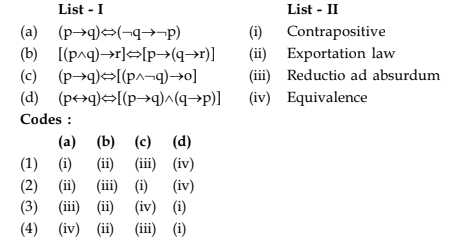UGC-NET | UGC NET CS 2015 Jun – II | Question 9

• Last Updated : 14 May, 2018

Match the following:(A) (1)
(B) (2)
(C) (3)
(D) (4)

Explanation:

• (p → q) ⇔ (¬q → ¬p) is Contrapositive
• [(p ∧ q) → r] ⇔ [p → (q → r)] is exportation law.
• (p → q) ⇔ [(p ∧ ¬q) → o] is Reductio ad absurdum
• (p ↔ q)⇔[(p → q)∧(q → p)] is Equivalence.

So, option (A) is correct.

Quiz of this Question

My Personal Notes arrow_drop_up